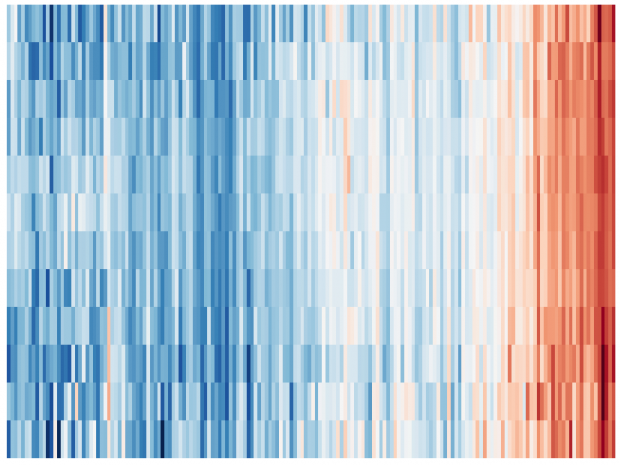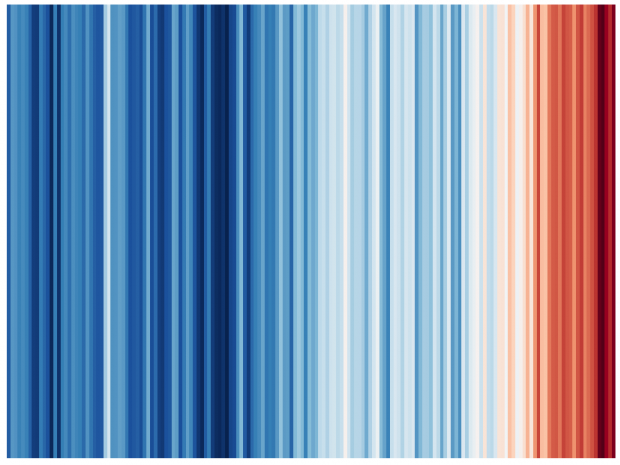# Correlation of earth temperature and global mean CO2

For teaching purposes I need the CO2 concentration vs earth temperature by year (Keeling curve). For that purpose we can use the Hadcrut 4 dataset created earlier while the global mean CO2 mix ratios (ppm) can be found at https://data.giss.nasa.gov/modelforce/ghgases/Fig1A.ext.txt.
After unscrambling that file and merging it to Hadcrut4 we can plot it

```library(patchwork)
p1 <- ggplot(temp, aes(x=year, y=annual)) + geom_point() + stat_smooth(method="loess", span = .6) +
scale_y_continuous( name="difference from baseline  [ oC ]", limits=c(-1,1) )

p2 <- ggplot(temp, aes(x=year, y=ppm )) +  geom_point() + stat_smooth(method="loess", span = .6) +
scale_y_continuous( name=expression('ppm CO') )
p1+p2
```

Here are the two time courses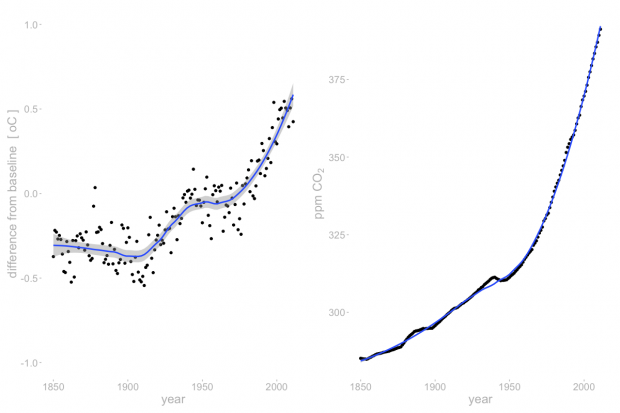Time course of earth temperature (1850-2018) and CO2 (1850-2011)

while the correlation is higher than I expected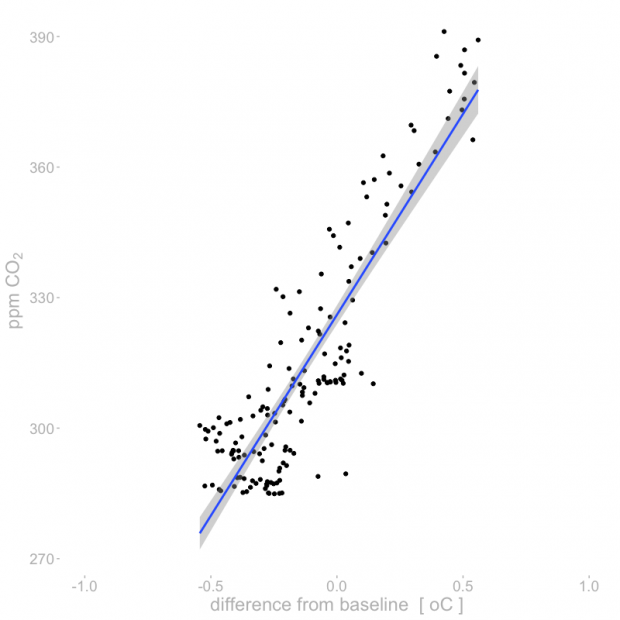Correlation of earth temperature and CO2

References

• 1850-1957: D.M. Etheridge, L.P. Steele, R.L. Langenfelds, R.J. Francey, J.-M. Barnola and V.I. Morgan, 1996, J. Geophys. Res., 101, 4115-4128,”Natural and anthroupogenic changes in atmospheric CO2 over the last 1000 years from air in Antarctic ice and firn”.
• 1958-1974: Means of Scripps Institution of Oceanography Continuous Data at Mauna Loa and South Pole provided by KenMaarie (personal communication)
• 1975-1982: Means of NOAA/CMDL in-situ data at Mauna Loa and South Pole. (P. Tans and K.W. Thoning, ftp://ftp.cmdl.noaa.gov/ccg/co2/in-situ)
• 1983-2003: Global means constructed using about 70 CMDL CCGG Sampling Network station data. (P.P. Tans and T.J. Conway, ftp://ftp.cmdl.noaa.gov/ccg/co2/flask)
• 2004-2007: Global mean growth rates. (T. Conway, ftp://ftp.cmdl.noaa.gov/ccg/co2/trends)

# A new animation of the famous HadCRUT4 climate dataset

Download

Here is the R sample code (PPT aspect ratio is 6:4, Youtube wants 16:9) .

As ggplot2 animation packages have major difficulties to manipulate the single frames, I am combining here raw PNGs using ffmpeg.

```# read_cru_hemi() modified from https://mccartneytaylor.com/plotting-climate-change-on-a-spider-graph-using-r

list.of.packages <- c("ggplot2", "reshape", "stringr","RColorBrewer")
new.packages <- list.of.packages[!(list.of.packages %in% installed.packages()[,"Package"])]
if(length(new.packages)) install.packages(new.packages)
lapply(list.of.packages, require, character.only = TRUE)

read_cru_hemi <- function(filename) {
tab <- read.table(filename,fill=TRUE)
nrows <- nrow(tab)
hemi <- data.frame(
year=tab[seq(1,nrows,2),1],
annual=tab[seq(1,nrows,2),14],
month=array(tab[seq(1,nrows,2),2:13]),
cover=array(tab[seq(2,nrows,2),2:13])
)
hemi[,15:26][ hemi[,15:26]==0 ] <- c(NA)
return(hemi)
}

url_dat <- "https://crudata.uea.ac.uk/cru/data/temperature/HadCRUT4-gl.dat"
tempdat <- read_cru_hemi(url_dat)
tempmelt <- melt(tempdat[,c(1,3:14)],id="year")

colfunc <- colorRampPalette(c("grey","grey","red"))
FadeToGrey <- colfunc(2019-1850)

new_theme <- theme_classic() + theme(
text = element_text(size=18, colour="grey"),
axis.line = element_blank(),
axis.text = element_text(colour="grey"),
axis.ticks = element_line(colour="grey"),
axis.title.x = element_blank(),
panel.border = element_blank(),
panel.grid.major = element_blank(),
panel.grid.minor = element_blank(),
panel.background = element_blank(),
legend.position = "none"
)
theme_set(new_theme)

for(i in 1850:2019){
p <- ggplot(tempmelt[tempmelt\$year %in% 1850:i,], aes(x=variable,y=value,color=as.factor(year),group=year)) +
geom_line() +
scale_x_discrete( labels=month.abb) +
scale_y_continuous( name="difference from baseline  [ oC ]", limits=c(-1,1) ) +
annotate("text", x=11, y=1, label=i, size=7) +
scale_color_manual( values=FadeToGrey[ 1:c(i-1849) ]  )
fn <- paste("/Users/xxx/Desktop/X/",str_pad(i-1849, 3, pad = "0"),".png",sep="")
ggsave(p, file=fn, width = 16, height = 9)
}

# not run
# ffmpeg -framerate 10 -i /Users/xxx/Desktop/X/%3d.png -r 5 -pix_fmt yuv420p -y /Users/xxx/Desktop/X/out.mp4
```

In comparison here is the original circular plot. Would require blue, green, yellow, red in the Color Ramp Palette…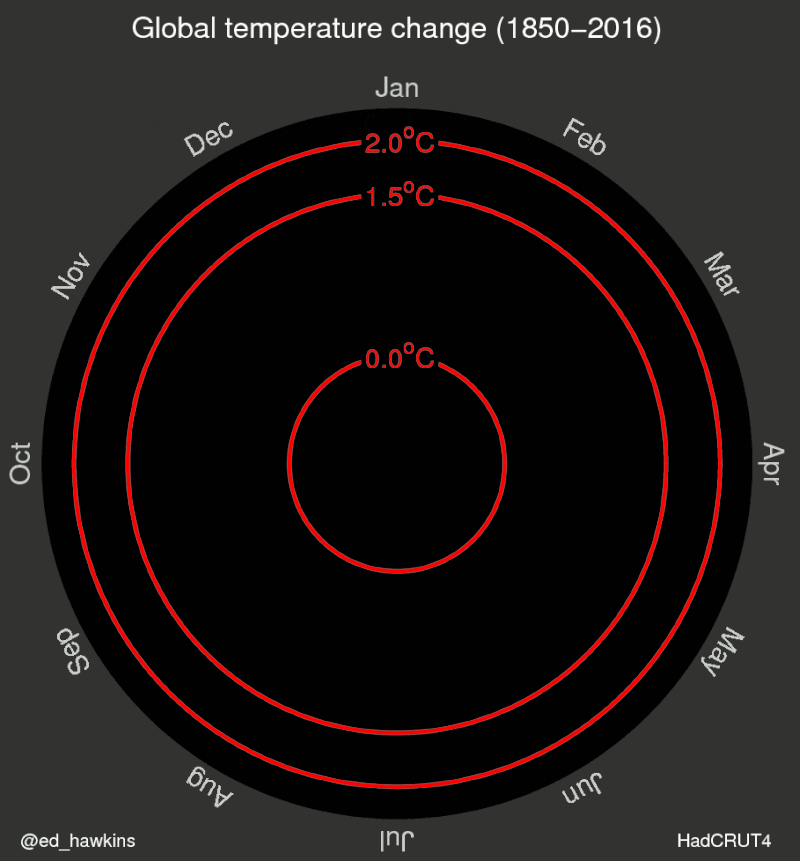Now it is only a minor step to the warming strips.

```ggplot(tempdat, aes(x = year, y = 1, fill = annual))+
geom_tile()+
scale_y_continuous(expand = c(0, 0))+
scale_x_continuous(expand = c(0, 0))+
scale_fill_gradientn(colors = rev(col_strip)) +
guides(fill = guide_colorbar(barwidth = 1)) +
theme( axis.ticks= element_blank(),
axis.text = element_blank(),
axis.title = element_blank()
)

tempmelt\$variable <- as.numeric(str_replace(as.character(tempmelt\$variable),"month.",""))
ggplot(tempmelt, aes(x = year, y = variable, z = value)) +
geom_raster(aes(fill = value)) +
scale_fill_gradientn(colors = rev(col_strip)) +
scale_x_continuous(expand = c(0,0)) +
scale_y_continuous(expand = c(0,0)) +
theme( axis.ticks= element_blank(),
axis.text = element_blank(),
axis.title = element_blank()
)
```